Ex 6.3

Chapter 6 Class 12 Application of Derivatives
Serial order wise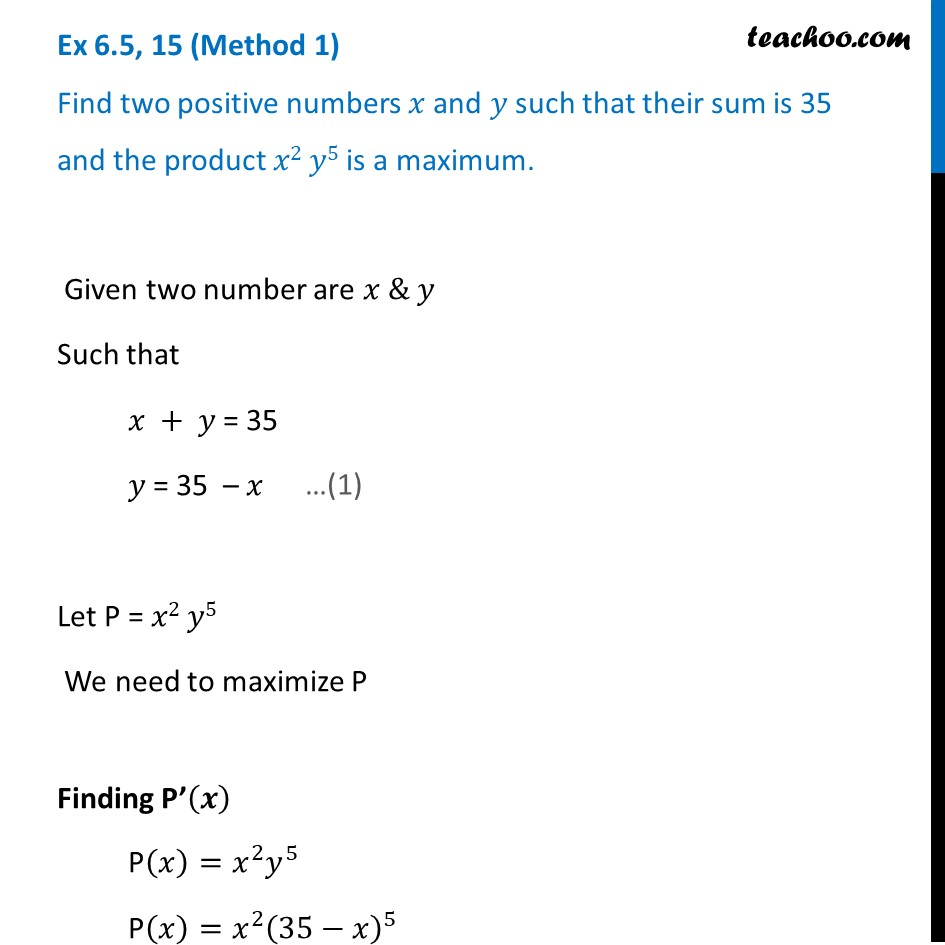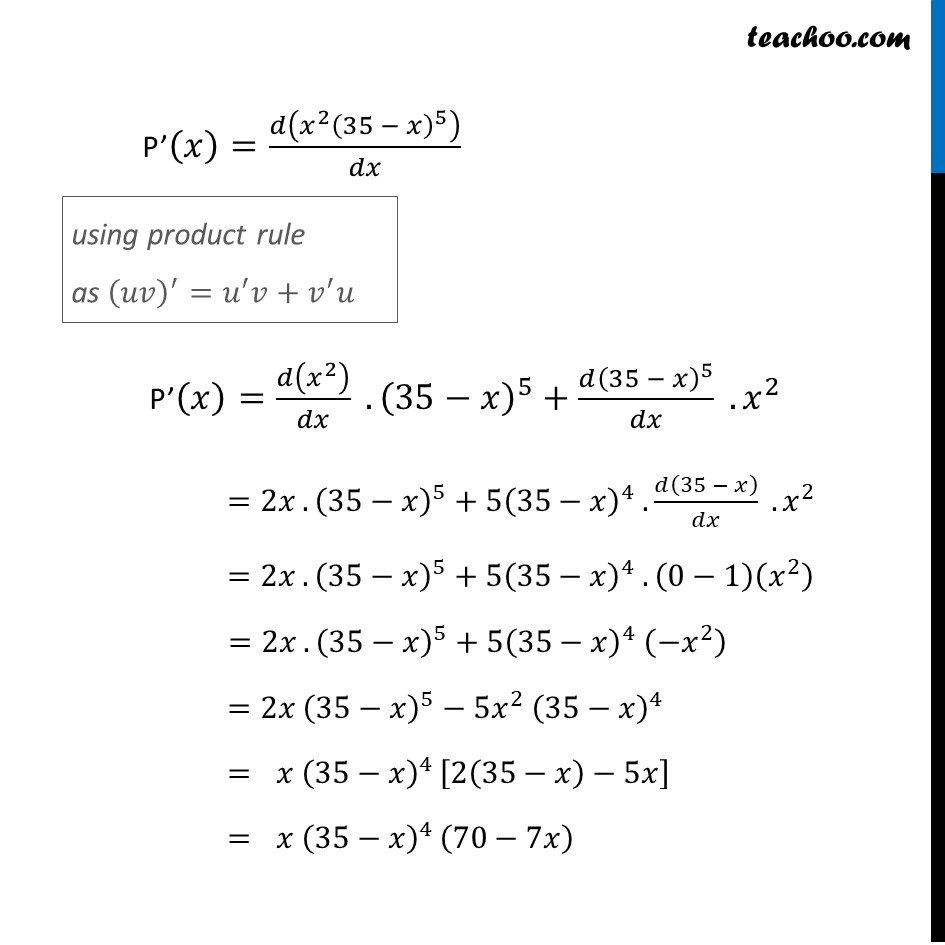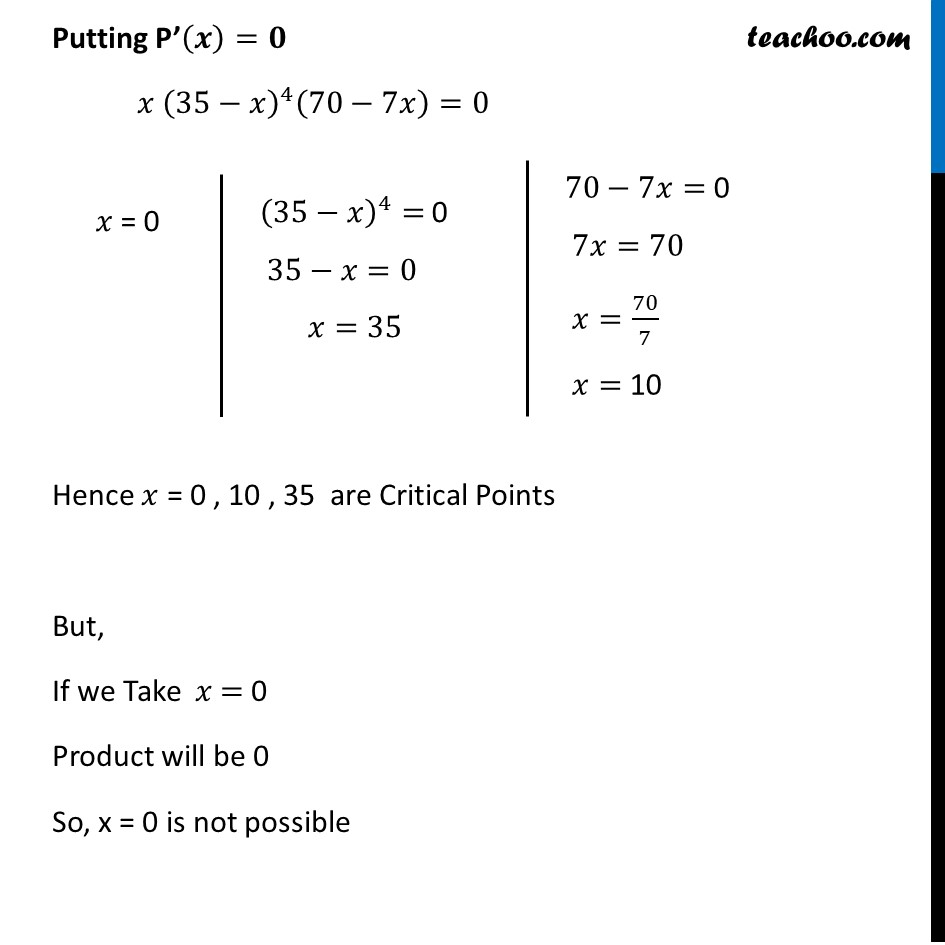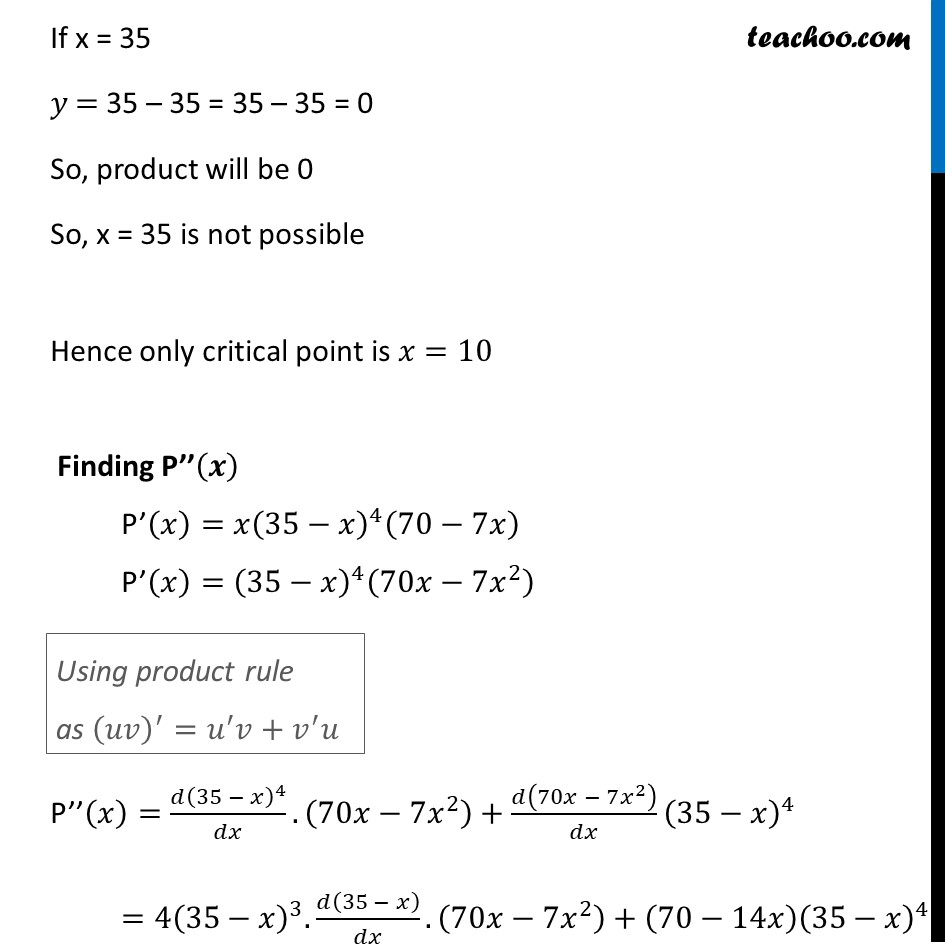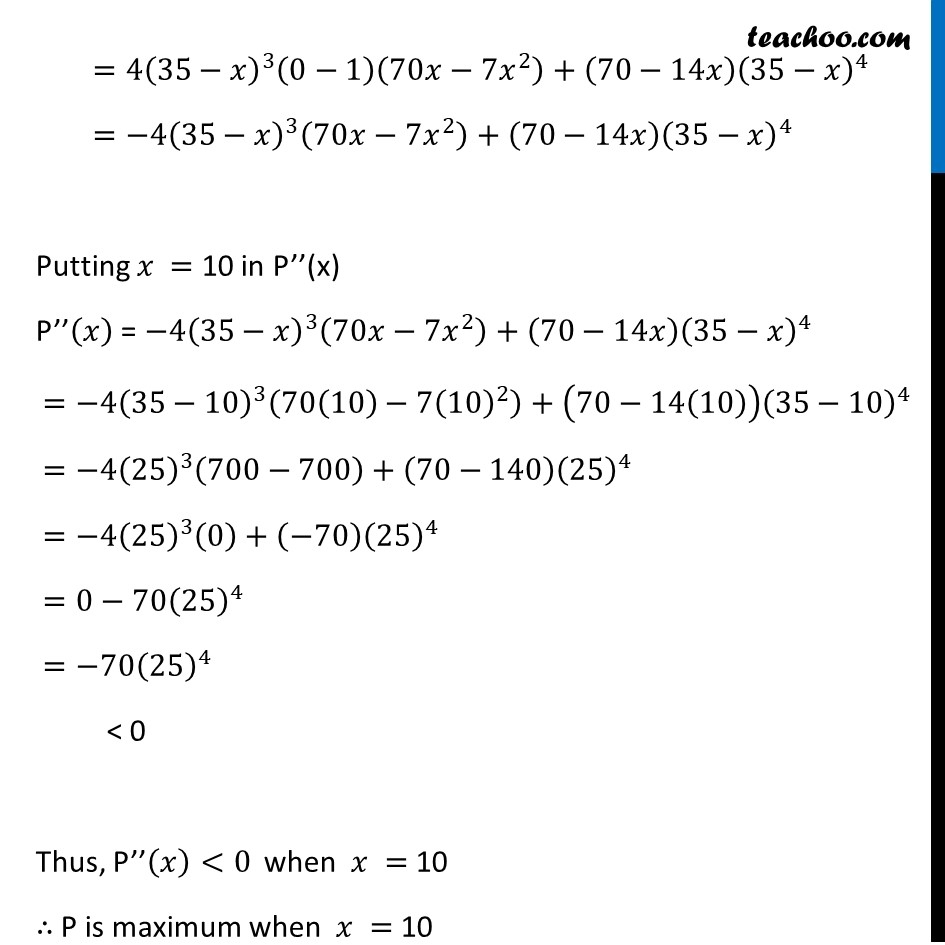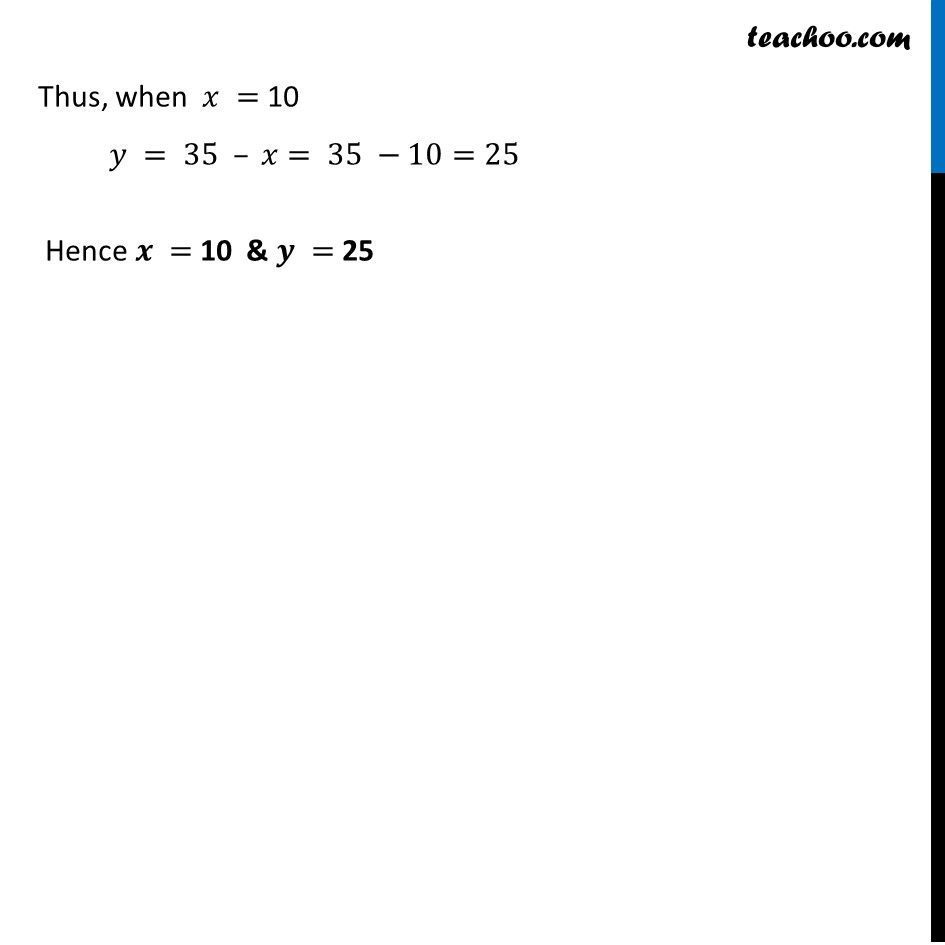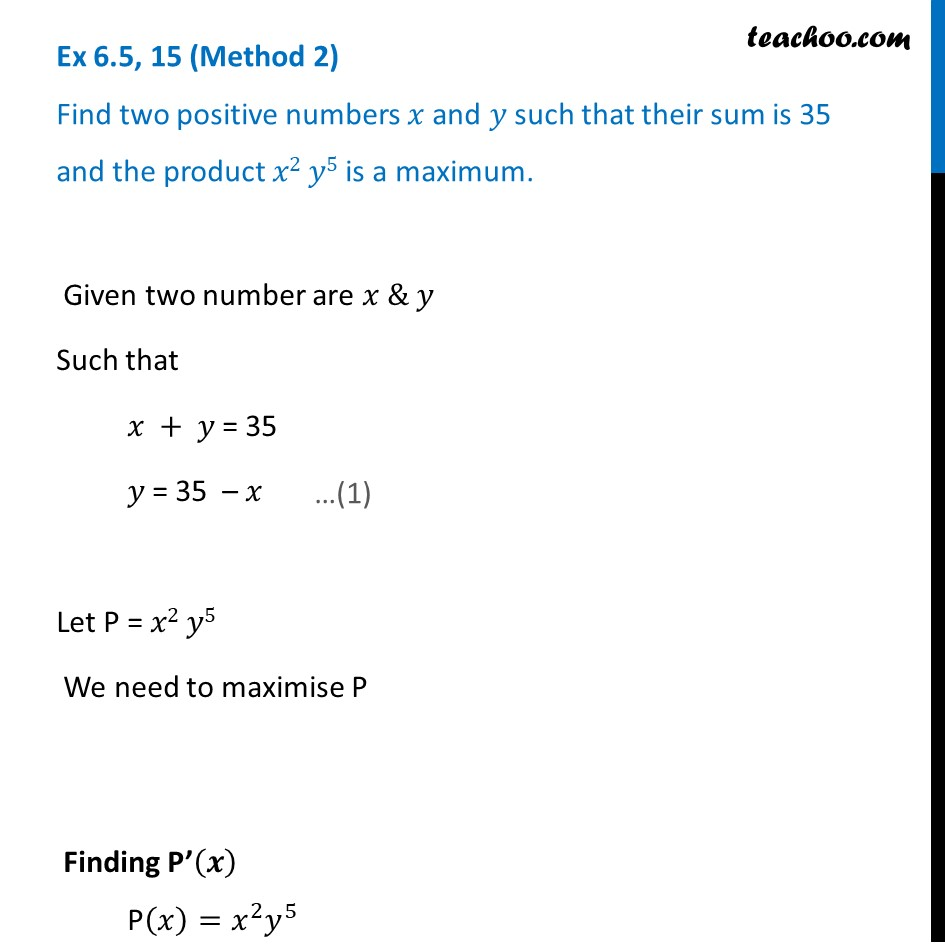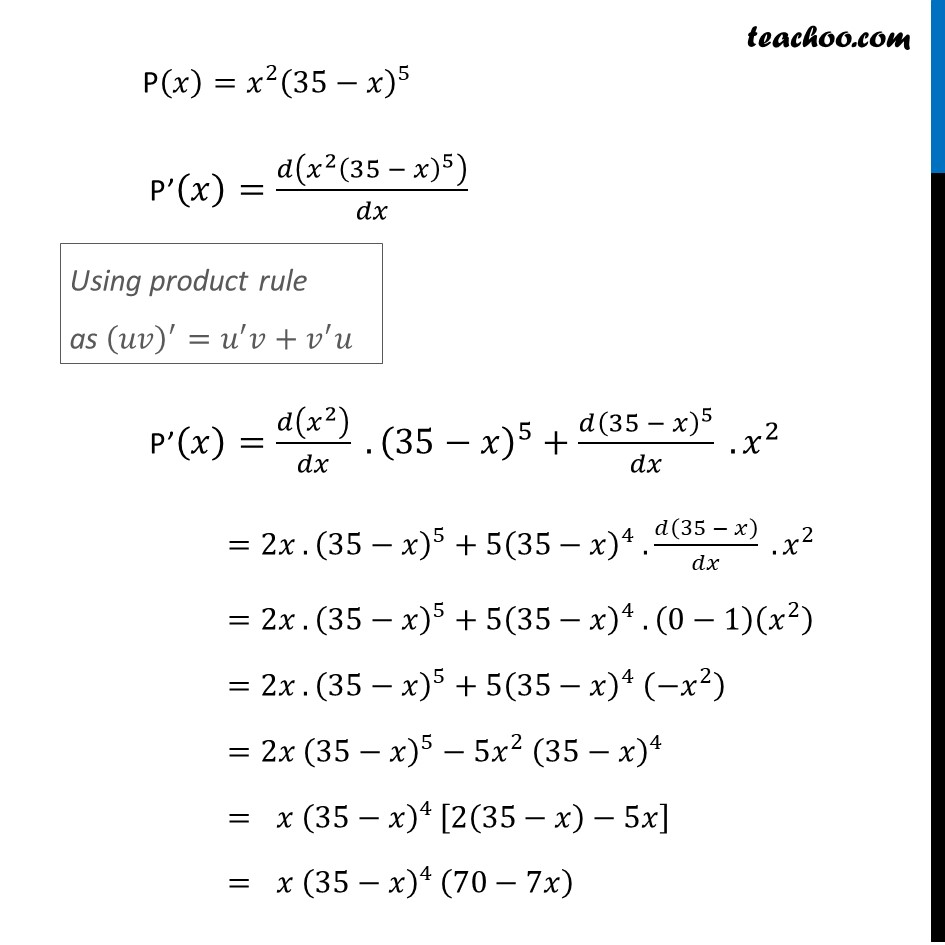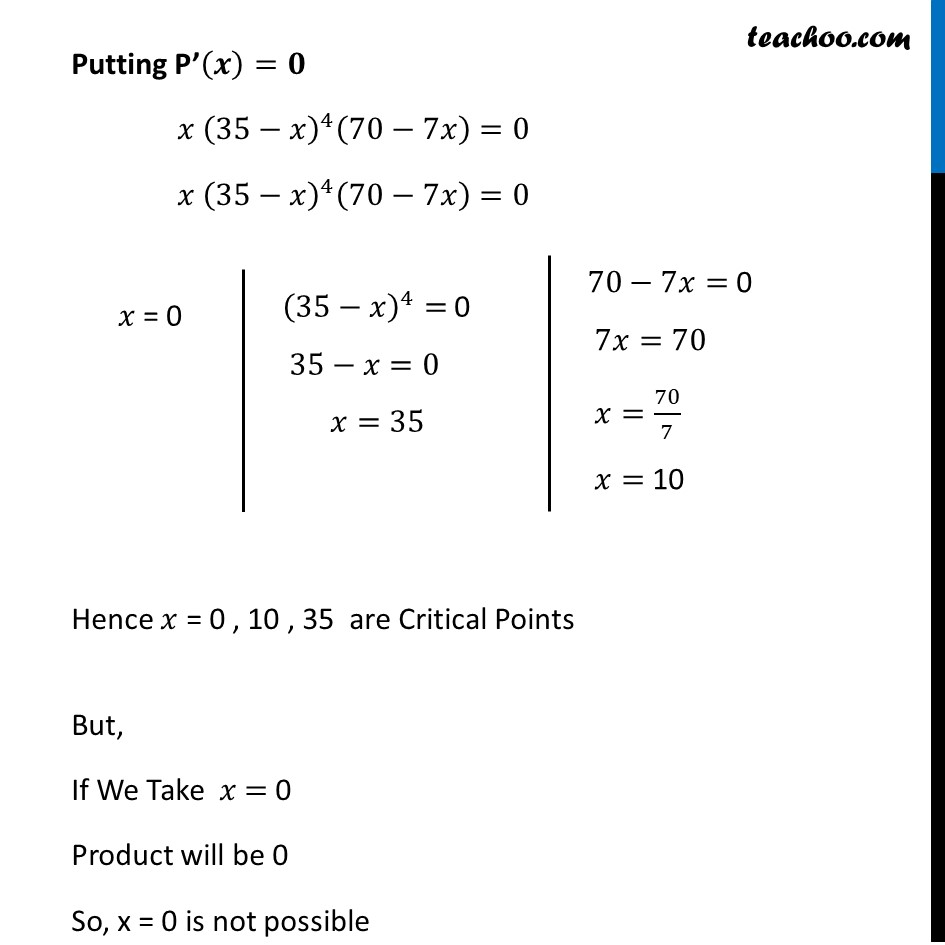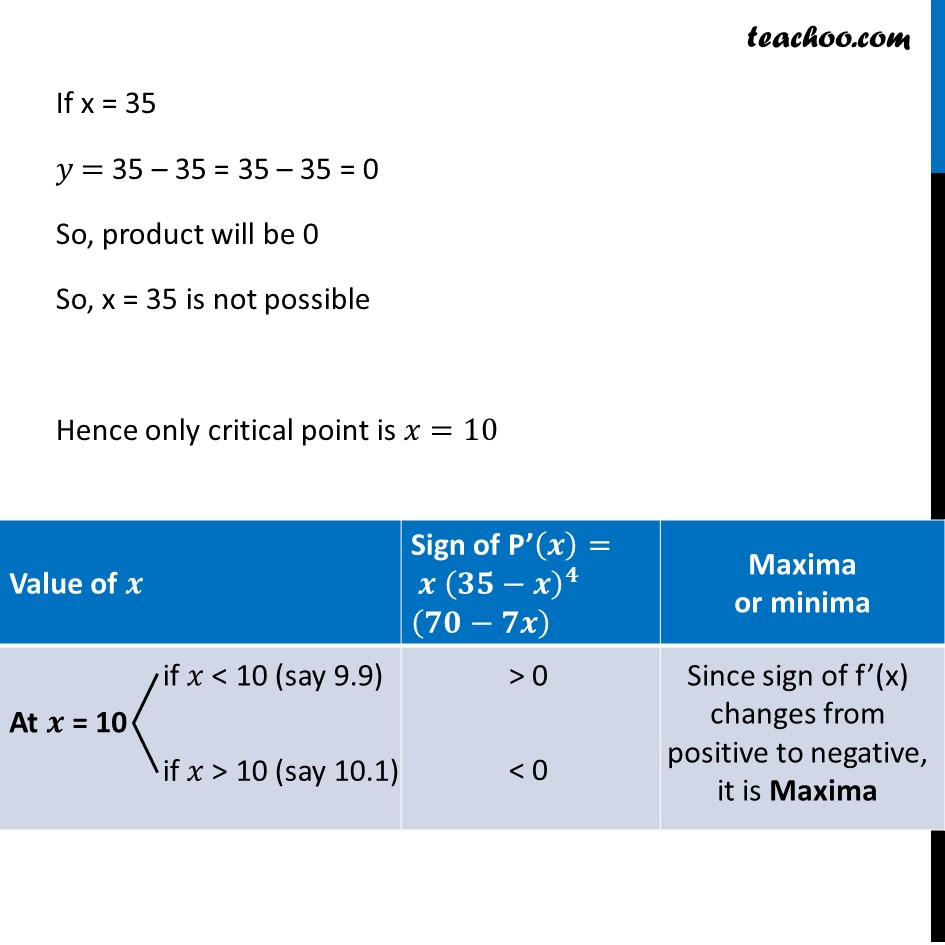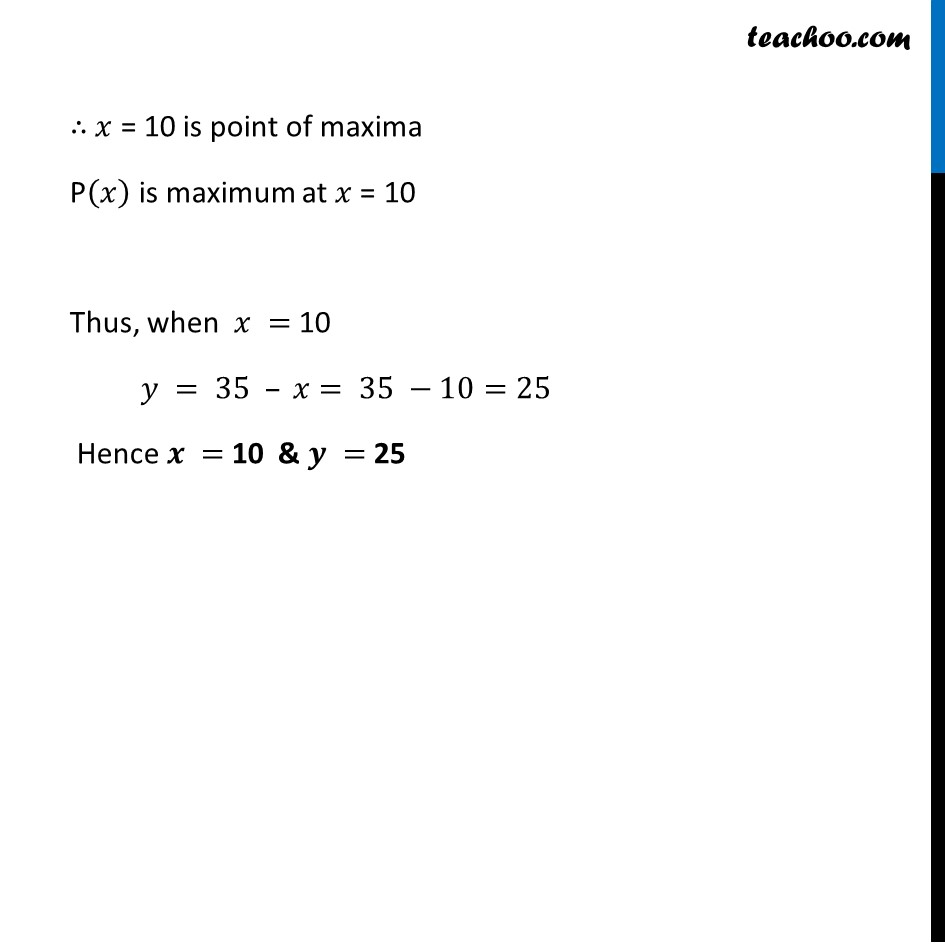Learn in your speed, with individual attention - Teachoo Maths 1-on-1 Class

### Transcript

Ex 6.3, 15 (Method 1) Find two positive numbers 𝑥 and 𝑦 such that their sum is 35 and the product 𝑥2 𝑦5 is a maximum. Given two number are 𝑥 & 𝑦 Such that 𝑥 + 𝑦 = 35 𝑦 = 35 – 𝑥 Let P = 𝑥2 𝑦5 We need to maximize P Finding P’(𝒙) P(𝑥)=𝑥^2 𝑦^5 P(𝑥)=𝑥^2 (35−𝑥)^5 P’(𝑥)=𝑑(𝑥^2 (35 − 𝑥)^5 )/𝑑𝑥 P’(𝑥)=𝑑(𝑥^2 )/𝑑𝑥 . (35−𝑥)^5+(𝑑(35 − 𝑥)^5)/𝑑𝑥 . 𝑥^2 =2𝑥 .(35−𝑥)^5+〖5(35−𝑥)〗^4 .𝑑(35 − 𝑥)/𝑑𝑥 . 𝑥^2 =2𝑥 .(35−𝑥)^5+〖5(35−𝑥)〗^4 . (0−1)(𝑥^2 ) =2𝑥 .(35−𝑥)^5+〖5(35−𝑥)〗^4 (−𝑥^2 ) =2𝑥 (35−𝑥)^5−〖5𝑥^2 (35−𝑥)〗^4 = 〖 𝑥 (35−𝑥)〗^4 [2(35−𝑥)−5𝑥] = 〖 𝑥 (35−𝑥)〗^4 (70−7𝑥) using product rule as (𝑢𝑣)^′=𝑢^′ 𝑣+𝑣^′ 𝑢 Putting P’(𝒙)=𝟎 〖 𝑥 (35−𝑥)〗^4 (70−7𝑥)=0 Hence 𝑥 = 0 , 10 , 35 are Critical Points But, If we Take 𝑥 = 0 Product will be 0 So, x = 0 is not possible (35−𝑥)^4= 0 35−𝑥=0 𝑥=35 70−7𝑥= 0 7𝑥=70 𝑥=70/7 𝑥= 10 If x = 35 𝑦 = 35 – 35 = 35 – 35 = 0 So, product will be 0 So, x = 35 is not possible Hence only critical point is 𝑥=10 Finding P’’(𝒙) P’(𝑥)=𝑥(35−𝑥)^4 (70−7𝑥) P’(𝑥)=(35−𝑥)^4 (70𝑥−7𝑥^2 ) P’’(𝑥)=(𝑑(35 − 𝑥)^4)/𝑑𝑥. (70𝑥−7𝑥^2 )+𝑑(70𝑥 − 7𝑥^2 )/𝑑𝑥 (35−𝑥)^4 =4(35−𝑥)^3.𝑑(35 − 𝑥)/𝑑𝑥. (70𝑥−7𝑥^2 )+(70−14𝑥) (35−𝑥)^4 Using product rule as (𝑢𝑣)^′=𝑢^′ 𝑣+𝑣^′ 𝑢 =4(35−𝑥)^3 (0−1)(70𝑥−7𝑥^2 )+(70−14𝑥) (35−𝑥)^4 =−4(35−𝑥)^3 (70𝑥−7𝑥^2 )+(70−14𝑥) (35−𝑥)^4 Putting 𝑥 = 10 in P’’(x) P’’(𝑥) = −4(35−𝑥)^3 (70𝑥−7𝑥^2 )+(70−14𝑥) (35−𝑥)^4 =−4(35−10)^3 (70(10)−7(10)^2 )+(70−14(10)) (35−10)^4 =−4(25)^3 (700−700)+(70−140) (25)^4 =−4(25)^3 (0)+(−70) (25)^4 =0−70(25)^4 =−70(25)^4 < 0 Thus, P’’(𝑥)<0 when 𝑥 = 10 ∴ P is maximum when 𝑥 = 10 Thus, when 𝑥 = 10 𝑦 = 35 – 𝑥= 35 −10=25 Hence 𝒙 = 10 & 𝒚 = 25 Ex 6.3, 15 (Method 2) Find two positive numbers 𝑥 and 𝑦 such that their sum is 35 and the product 𝑥2 𝑦5 is a maximum. Given two number are 𝑥 & 𝑦 Such that 𝑥 + 𝑦 = 35 𝑦 = 35 – 𝑥 Let P = 𝑥2 𝑦5 We need to maximise P Finding P’(𝒙) P(𝑥)=𝑥^2 𝑦^5 P(𝑥)=𝑥^2 (35−𝑥)^5 P’(𝑥)=𝑑(𝑥^2 (35 − 𝑥)^5 )/𝑑𝑥 P’(𝑥)=𝑑(𝑥^2 )/𝑑𝑥 . (35−𝑥)^5+(𝑑(35 − 𝑥)^5)/𝑑𝑥 . 𝑥^2 =2𝑥 .(35−𝑥)^5+〖5(35−𝑥)〗^4 .𝑑(35 − 𝑥)/𝑑𝑥 . 𝑥^2 =2𝑥 .(35−𝑥)^5+〖5(35−𝑥)〗^4 . (0−1)(𝑥^2 ) =2𝑥 .(35−𝑥)^5+〖5(35−𝑥)〗^4 (−𝑥^2 ) =2𝑥 (35−𝑥)^5−〖5𝑥^2 (35−𝑥)〗^4 = 〖 𝑥 (35−𝑥)〗^4 [2(35−𝑥)−5𝑥] = 〖 𝑥 (35−𝑥)〗^4 (70−7𝑥) Using product rule as (𝑢𝑣)^′=𝑢^′ 𝑣+𝑣^′ 𝑢 Putting P’(𝒙)=𝟎 〖𝑥 (35−𝑥)〗^4 (70−7𝑥)=0 〖𝑥 (35−𝑥)〗^4 (70−7𝑥)=0 Hence 𝑥 = 0 , 10 , 35 are Critical Points But, If We Take 𝑥 = 0 Product will be 0 So, x = 0 is not possible (35−𝑥)^4= 0 35−𝑥=0 𝑥=35 70−7𝑥= 0 7𝑥=70 𝑥=70/7 𝑥= 10 If x = 35 𝑦 = 35 – 35 = 35 – 35 = 0 So, product will be 0 So, x = 35 is not possible Hence only critical point is 𝑥=10 ∴ 𝑥 = 10 is point of maxima P(𝑥) is maximum at 𝑥 = 10 Thus, when 𝑥 = 10 𝑦 = 35 – 𝑥= 35 −10=25 Hence 𝒙 = 10 & 𝒚 = 25